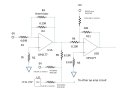# Op Amp Circuit - Summing Amplifier - Are these Equations correct?

#### matthej

Joined Oct 10, 2020
66
Hi,

I am trying to figure out the equations for the following circuit. First of all U1a is attached to a thermistor, so it looks like the Gain of the circuit will be proportional to the value of the thermistor (Vout/Vin = -Rf/Ri).

After that, for U1B, I am trying to figure out what is going on. It looks like its a summing amplifier?
So: Vout = (-Rf/Rin1)V1 + (-Rf/Rin2)V2 etc?

So would it be:

Vout = -(10K/R9+R10)(-5V) + -(10K/R4+R6+R7+R8)(-5V) + -(10K/R4+R6+R12+R13)(DAC value) + -(10K/R4)(U1A value)?

Does this look correct?

Thanks!#### Audioguru again

Joined Oct 21, 2019
6,425
The output impedance of U1A will be so low that varying current in R6 will have almost no effect on the output of U1B.

#### AnalogKid

Joined Aug 1, 2013
10,763

ak